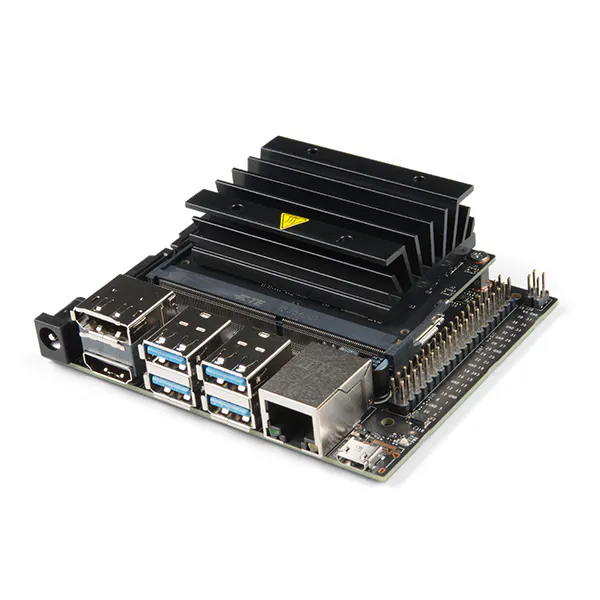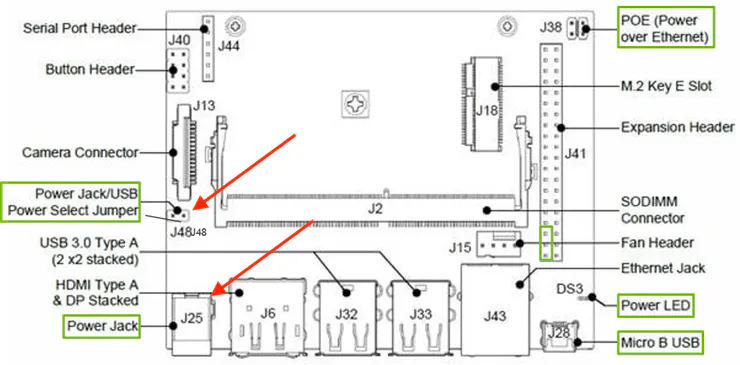Created February 11, 2020 © GPL3+

# Snore AI

Using Jetson Nano to monitor a good night sleep

AdvancedFull instructions provided20 hours173## Things used in this project

### Hardware componentsNVIDIA Jetson Nano Developer Kit
×1Microphone, Plug-In
×1

### Software apps and online services

 NVIDIA Jetson Jetpack

### Hand tools and fabrication machines3D Printer (generic)

Read more

## Schematics

### Jetson Nano Schematic

Jetson Nano Schematic## Code

### snore_detect.py

Python
Snore AI run file, please pass the model and label file
```from collections import deque
import logging
import os
import subprocess
import threading
import time
import wave
import tensorflow as tf
import numpy as np
from datetime import datetime
import pyaudio
import wave
import audioop
import json

isRecording = False
currentLabel = ''
# pylint: disable=unused-import
from tensorflow.contrib.framework.python.ops import audio_ops as contrib_audio
# pylint: enable=unused-import

logger = logging.getLogger('audio')

def sample_width_to_string(sample_width):
"""Convert sample width (bytes) to ALSA format string."""
return {1: 's8', 2: 's16', 4: 's32'}[sample_width]

class Recorder(threading.Thread):
"""Stream audio from microphone in a background thread and run processing
callbacks. It reads audio in a configurable format from the microphone,
then converts it to a known format before passing it to the processors.
"""
CHUNK_S = 0.1

def __init__(self,
input_device='default',
channels=1,
bytes_per_sample=2,
sample_rate_hz=16000):
"""Create a Recorder with the given audio format.
The Recorder will not start until start() is called. start() is called
automatically if the Recorder is used in a `with`-statement.
- input_device: name of ALSA device (for a list, run `arecord -L`)
- channels: number of channels in audio read from the mic
- bytes_per_sample: sample width in bytes (eg 2 for 16-bit audio)
- sample_rate_hz: sample rate in hertz
"""

super(Recorder, self).__init__()

self._processors = []

self._chunk_bytes = int(
self.CHUNK_S * sample_rate_hz) * channels * bytes_per_sample

self._cmd = [
'arecord',
'-q',
'-t',
'raw',
'-D',
input_device,
'-c',
str(channels),
'-f',
sample_width_to_string(bytes_per_sample),
'-r',
str(sample_rate_hz),
]
self._arecord = None
self._closed = False

def add_processor(self, processor):
self._processors.append(processor)

def del_processor(self, processor):
self._processors.remove(processor)

def run(self):
"""Reads data from arecord and passes to processors."""
global isRecording
global currentLabel
recordCounter = 0
sample_format = pyaudio.paInt16  # 16 bits per sample
channels = 1
fs = 16000  # Record at 16000 samples per second
seconds = 3
filename = "tmp/output.wav"
p = pyaudio.PyAudio()  # Create an interface to PortAudio
stream = p.open(format=sample_format,
channels=channels,
rate=fs,
frames_per_buffer=self._chunk_bytes,
input=True)
self._arecord = subprocess.Popen(self._cmd, stdout=subprocess.PIPE)
logger.info('started recording')

# check for race-condition when __exit__ is called at the same time as
# the process is started by the background thread
if self._closed:
self._arecord.kill()
return

this_chunk = b''
frames = []  # Initialize array to store frames

while True:
input_data = self._arecord.stdout.read(self._chunk_bytes)
if not input_data:
break

this_chunk += input_data
rms = audioop.rms(this_chunk, 2)    # here's where you calculate the volume
starttime = datetime.now().strftime("%m-%d-%Y+%H:%M:%S")
#start recording
if isRecording == True and recordCounter == 0:
starttime = datetime.now().strftime("%m-%d-%Y+%H:%M:%S")
filename = "tmp/"+ datetime.now().strftime("%m-%d-%Y+%H:%M:%S") + ".wav"
data = stream.read(self._chunk_bytes)
frames.append(data)
recordCounter += 1
print("start recording" + filename)
#stops recording
elif isRecording == True and recordCounter > 100 and rms < 150:
isRecording = False
recordCounter = 0
# Save the recorded data as a WAV file
wf = wave.open(filename, 'wb')
wf.setnchannels(channels)
wf.setsampwidth(p.get_sample_size(sample_format))
wf.setframerate(fs)
wf.writeframes(b''.join(frames))
duration = wf.getnframes() / float(wf.getframerate())
wf.close()

print("stop recording" + filename)
with open('tmp/data.txt') as json_file:
data = json.load(json_file)
data['data'].append(
{
'time': starttime,
'file': filename,
'label': currentLabel,
'duration': duration
}
)
with open('tmp/data.txt', 'w') as outfile:
json.dump(data, outfile)
frames = []

#still noise
elif isRecording == True and recordCounter > 100 and rms > 150:
data = stream.read(self._chunk_bytes)
frames.append(data)
recordCounter = 1

#keeps recording
elif isRecording == True:
recordCounter += 1
data = stream.read(self._chunk_bytes)
frames.append(data)
print(str(rms) + "," + datetime.now().strftime("%m-%d-%Y+%H:%M:%S"))

if len(this_chunk) >= self._chunk_bytes:
self._handle_chunk(this_chunk[:self._chunk_bytes])
this_chunk = this_chunk[self._chunk_bytes:]

if not self._closed:
logger.error('Microphone recorder died unexpectedly, aborting...')
# sys.exit doesn't work from background threads, so use os._exit as
# an emergency measure.
logging.shutdown()
os._exit(1)  # pylint: disable=protected-access

def _handle_chunk(self, chunk):
"""Send audio chunk to all processors.
"""
for p in self._processors:
p.add_data(chunk)

def __enter__(self):
self.start()
return self

def __exit__(self, *args):
self._closed = True
if self._arecord:
self._arecord.kill()

class RecognizePredictions(object):

def __init__(self, time, predictions):
self.time_ = time
self.predictions_ = predictions

def time(self):
return self.time_

def predictions(self):
return self.predictions_

class RecognizeCommands(object):
"""A processor that identifies spoken commands from the stream."""

def __init__(self, graph, labels, input_samples_name, input_rate_name,
output_name, average_window_duration_ms, detection_threshold,
suppression_ms, minimum_count, sample_rate, sample_duration_ms):
self.input_samples_name_ = input_samples_name
self.input_rate_name_ = input_rate_name
self.output_name_ = output_name
self.average_window_duration_ms_ = average_window_duration_ms
self.detection_threshold_ = detection_threshold
self.suppression_ms_ = suppression_ms
self.minimum_count_ = minimum_count
self.sample_rate_ = sample_rate
self.sample_duration_ms_ = sample_duration_ms
self.previous_top_label_ = '_silence_'
self.previous_top_label_time_ = 0
self.recording_length_ = int((sample_rate * sample_duration_ms) / 1000)
self.recording_buffer_ = np.zeros(
[self.recording_length_], dtype=np.float32)
self.recording_offset_ = 0
self.sess_ = tf.Session()
self._load_graph(graph)
self.labels_ = self._load_labels(labels)
self.labels_count_ = len(self.labels_)
self.previous_results_ = deque()

def _load_graph(self, filename):
"""Unpersists graph from file as default graph."""
with tf.gfile.FastGFile(filename, 'rb') as f:
graph_def = tf.GraphDef()
graph_def.ParseFromString(f.read())
tf.import_graph_def(graph_def, name='')

def _load_labels(self, filename):
"""Read in labels, one label per line."""
return [line.rstrip() for line in tf.gfile.GFile(filename)]

def add_data(self, data_bytes):
global isRecording
global currentLabel
"""Process audio data."""
if not data_bytes:
return
data = np.frombuffer(data_bytes, dtype=np.int16)
current_time_ms = int(round(time.time() * 1000))
number_read = len(data)
new_recording_offset = self.recording_offset_ + number_read
second_copy_length = max(0, new_recording_offset - self.recording_length_)
first_copy_length = number_read - second_copy_length
self.recording_buffer_[self.recording_offset_:(
self.recording_offset_ + first_copy_length
)] = data[:first_copy_length].astype(np.float32) * (1 / 32767.0)
self.recording_buffer_[:second_copy_length] = data[
first_copy_length:].astype(np.float32) * (1 / 32767.0)
self.recording_offset_ = new_recording_offset % self.recording_length_
input_data = np.concatenate(
(self.recording_buffer_[self.recording_offset_:],
self.recording_buffer_[:self.recording_offset_]))
input_data = input_data.reshape([self.recording_length_, 1])
softmax_tensor = self.sess_.graph.get_tensor_by_name(self.output_name_)
predictions, = self.sess_.run(softmax_tensor, {
self.input_samples_name_: input_data,
self.input_rate_name_: self.sample_rate_
})
if self.previous_results_ and current_time_ms < self.previous_results_.time(
):
raise RuntimeException(
'You must feed results in increasing time order, but received a '
'timestamp of ', current_time_ms,
' that was earlier than the previous one of ',
self.previous_results_.time())

self.previous_results_.append(
RecognizePredictions(current_time_ms, predictions))
# Prune any earlier results that are too old for the averaging window.
time_limit = current_time_ms - self.average_window_duration_ms_
while self.previous_results_.time() < time_limit:
self.previous_results_.popleft()
# If there are too few results, assume the result will be unreliable and
# bail.
how_many_results = len(self.previous_results_)
earliest_time = self.previous_results_.time()
samples_duration = current_time_ms - earliest_time
if how_many_results < self.minimum_count_ or samples_duration < (
self.average_window_duration_ms_ / 4):
return

# Calculate the average score across all the results in the window.
average_scores = np.zeros([self.labels_count_])
for result in self.previous_results_:
average_scores += result.predictions() * (1.0 / how_many_results)

# Sort the averaged results in descending score order.
top_result = average_scores.argsort()[-1:][::-1]

# See if the latest top score is enough to trigger a detection.
current_top_index = top_result
current_top_label = self.labels_[current_top_index]
current_top_score = average_scores[current_top_index]
# If we've recently had another label trigger, assume one that occurs too
# soon afterwards is a bad result.
if self.previous_top_label_ == '_silence_' or self.previous_top_label_time_ == 0:
time_since_last_top = 1000000
else:
time_since_last_top = current_time_ms - self.previous_top_label_time_

if current_top_score > self.detection_threshold_ and time_since_last_top > self.suppression_ms_:
self.previous_top_label_ = current_top_label
self.previous_top_label_time_ = current_time_ms
is_new_command = True
logger.info(current_top_label)
currentLabel = current_top_label
isRecording = True
else:
is_new_command = False

def is_done(self):
return False

def __enter__(self):
return self

def __exit__(self, *args):
pass

def main():
logging.basicConfig(level=logging.INFO)

import argparse
import time

parser = argparse.ArgumentParser(description='Test audio wrapper')
parser.add_argument(
'-I',
'--input-device',
default='default',
help='Name of the audio input device')
parser.add_argument(
'-c', '--channels', type=int, default=1, help='Number of channels')
parser.add_argument(
'-f',
'--bytes-per-sample',
type=int,
default=2,
help='Sample width in bytes')
parser.add_argument(
'-r', '--rate', type=int, default=16000, help='Sample rate in Hertz')
parser.add_argument(
'--graph', type=str, default='', help='Model to use for identification.')
parser.add_argument(
'--labels', type=str, default='', help='Path to file containing labels.')
parser.add_argument(
'--input_samples_name',
type=str,
default='decoded_sample_data:0',
help='Name of PCM sample data input node in model.')
parser.add_argument(
'--input_rate_name',
type=str,
default='decoded_sample_data:1',
help='Name of sample rate input node in model.')
parser.add_argument(
'--output_name',
type=str,
default='labels_softmax:0',
help='Name of node outputting a prediction in the model.')
parser.add_argument(
'--average_window_duration_ms',
type=int,
default='500',
help='How long to average results over.')
parser.add_argument(
'--detection_threshold',
type=float,
default='0.6',
help='Score required to trigger recognition.')
parser.add_argument(
'--suppression_ms',
type=int,
default='1500',
help='How long to ignore recognitions after one has triggered.')
parser.add_argument(
'--minimum_count',
type=int,
default='2',
help='How many recognitions must be present in a window to trigger.')
parser.add_argument(
'--sample_rate', type=int, default='16000', help='Audio sample rate.')
parser.add_argument(
'--sample_duration_ms',
type=int,
default='1000',
help='How much audio the recognition model looks at.')
args = parser.parse_args()

recorder = Recorder(
input_device=args.input_device,
channels=args.channels,
bytes_per_sample=args.bytes_per_sample,
sample_rate_hz=args.rate)

recognizer = RecognizeCommands(
args.graph, args.labels, args.input_samples_name, args.input_rate_name,
args.output_name, args.average_window_duration_ms,
args.detection_threshold, args.suppression_ms, args.minimum_count,
args.sample_rate, args.sample_duration_ms)

with recorder, recognizer:
recorder.add_processor(recognizer)
while not recognizer.is_done():
time.sleep(0.03)

if __name__ == '__main__':
main()
```

### wave_to_spectrogram

Python
changing wav file into spectrogram
```import tensorflow as tf
# FIXME: audio_ops.decode_wav is deprecated, use tensorflow_io.IOTensor.from_audio
from tensorflow.contrib.framework.python.ops import audio_ops

# Enable eager execution for a more interactive frontend.
# If using the default graph mode, you'll probably need to run in a session.
tf.enable_eager_execution()

@tf.function
def audio_to_spectrogram(
audio_contents,
width,
height,
channels=1,
window_size=1024,
stride=64,
brightness=100.):
"""Decode and build a spectrogram using a wav string tensor.

Args:
audio_contents: String tensor of the wav audio contents.
width: Spectrogram width.
height: Spectrogram height.
channels: Audio channel count.
window_size: Size of the spectrogram window.
stride: Size of the spectrogram stride.
brightness: Brightness of the spectrogram.

Returns:
0-D string Tensor with the image contents.
"""
# Decode the wav mono into a 2D tensor with time in dimension 0
# and channel along dimension 1
waveform = audio_ops.decode_wav(audio_contents, desired_channels=channels)

# Compute the spectrogram
# FIXME: Seems like this is deprecated in tensorflow 2.0 and
# the operation only works on CPU. Change this to tf.signal.stft
# and  friends to take advantage of GPU kernels.
spectrogram = audio_ops.audio_spectrogram(
waveform.audio,
window_size=window_size,
stride=stride)

# Adjust brightness
brightness = tf.constant(brightness)

# Normalize pixels
mul = tf.multiply(spectrogram, brightness)
min_const = tf.constant(255.)
minimum = tf.minimum(mul, min_const)

# Expand dims so we get the proper shape
expand_dims = tf.expand_dims(minimum, -1)

# Resize the spectrogram to input size of the model
resize = tf.image.resize(expand_dims, [width, height])

# Remove the trailing dimension
squeeze = tf.squeeze(resize, 0)

# Tensorflow spectrogram has time along y axis and frequencies along x axis
# so we fix that
flip_left_right = tf.image.flip_left_right(squeeze)
transposed = tf.image.transpose(flip_left_right)

# Cast to uint8 and encode as png
cast = tf.cast(transposed, tf.uint8)

# Encode tensor as a png image
return tf.image.encode_png(cast)

if __name__ == '__main__':
input_file = tf.constant('record.wav')
output_file = tf.constant('spectrogram.png')

# Generage the spectrogram
audio = tf.io.read_file(input_file)
image = audio_to_spectrogram(audio, 224, 224)

# Write the png encoded image to a file
tf.io.write_file(output_file, image)
```

### SnoreAI repo

Snore AI github repo

## Credits

### Peter Ma

49 projects • 348 followers
Prototype Hacker, Hackathon Goer, World Traveler, Ecological balancer, integrationist, technologist, futurist.

### Ethan Fan

9 projects • 29 followers
Mobile, Watch, and Glass Developer

### Stephanie Ko

2 projects • 10 followers
Mobile & IOT developer